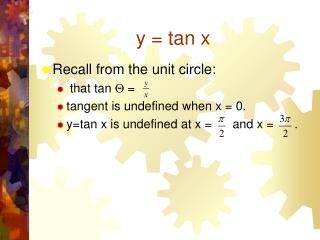Download Presentationy = tan x

Loading in 2 Seconds...

# y = tan x - PowerPoint PPT Presentation

y = tan x. Recall from the unit circle: that tan  = tangent is undefined when x = 0. y=tan x is undefined at x = and x =. Domain/Range of the Tangent Function. The tangent function is undefined at + k  . Asymptotes are at every multiple of + k  .I am the owner, or an agent authorized to act on behalf of the owner, of the copyrighted work described.
Download Presentation## y = tan x

An Image/Link below is provided (as is) to download presentation

Download Policy: Content on the Website is provided to you AS IS for your information and personal use and may not be sold / licensed / shared on other websites without getting consent from its author.While downloading, if for some reason you are not able to download a presentation, the publisher may have deleted the file from their server.

- - - - - - - - - - - - - - - - - - - - - - - - - - E N D - - - - - - - - - - - - - - - - - - - - - - - - - -
Presentation Transcript
1. y = tan x • Recall from the unit circle: • that tan  = • tangent is undefined when x = 0. • y=tan x is undefined at x = and x = .

2. Domain/Range of the Tangent Function • The tangent function is undefined at + k. • Asymptotes are at every multiple of + k . • The domain is (-,  except + k ). • Graphs must contain the dotted asymptote lines. These lines will move if the function contains a horizontal shift, stretch or shrink. • The range of every tan graph is (-, ).

3. Period of Tangent Function • This also means that one complete cycle occurs between and . • The period is .

4. Critical Points • The range is unlimited; there is no maximum. • The range is unlimited; there is no minimum.

5. y = tan x Key Points • : asymptote. The graph approaches - as it near this asymptote • ( , -1), (0,0), (, 1) • : asymptote. The graph approaches  as it nears this asymptote

6. Graph of the Parent Function

7. Parent Function: (-,)

8. The Graph: y = a tan b (x - c)+ d • a = vertical stretch or shrink • If |a| > 1, there is a vertical stretch. • If 0<|a|<1, there is a vertical shrink. • If a is negative, the graph reflects about the x-axis.

9. y = 4 tan x

10. y = a tan b (x - c) + d • b= horizontal stretch or shrink • Period = • If |b| > 1, there is a horizontal shrink. • If 0 < |b| < 1, there is a horizontal stretch. • If b<0, the graph reflects about the y-axis.

11. y = tan 2x

12. y = a tan b (x - c) + d • c = horizontal shift • If c is negative, the graph shifts left c units. (x - (-c)) = (x + c) • If c is positive, the graph shifts right c units. (x - (+c)) = (x - c)

13. y = tan (x - /2)

14. y = a tan b (x-c) + d • d= vertical shift • If d is positive, graph shifts up d units. • If d is negative, graph shifts down d units.

15. y = tan x + 3

16. y = 3 tan (2x-) - 3